# Dosage calculationEnsuring effective therapy

Dosage calculation
Ensuring effective therapy

Calculating drug dosages

Reviewing ratios and proportions

A ratio is a mathematical expression of the relationship between two things. A proportion is a set of two equal ratios. A ratio may be expressed as a fraction, such as 1/3, or with a colon, such as 1:3. When ratios are expressed as fractions in a proportion, their cross products are equal.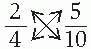When ratios are expressed using colons in a proportion, the product of the means equals the product of the extremes.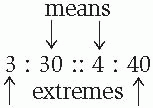Product of means and extremes

30 × 4 = 3 × 40

Whether fractions or ratios are used in a proportion, they must appear in the same order on both sides of the equal sign. When ratios are expressed as fractions, the units in the numerators must be the same and the units in the denominators must be the same (although they don’t have to be the same as the units in the numerators).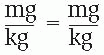If the ratios in a proportion are expressed with colons, the units of the first term on the left side of the equal sign must be the same as the units of the first term on the right side. In other words, the units of the mean on one side of the equal sign must match the units of the extreme on the other side, and vice versa.

mg : kg :: mg : kg

Tips for simplifying dosage calculations

Incorporate units of measure into the calculation

Incorporating units of measure into the dosage calculation helps to protect you from one of the most common errors made in dosage calculation—using the incorrect unit of measure. Keep in mind that the units of measure that appear in the numerator and denominator cancel each other out, leaving the correct unit of measure in the answer. The following example uses units of measure in calculating a drug with a usual dose of 4 mg/kg for a 55-kg patient.

1. State the problem as a proportion.

4 mg: 1 kg :: X : 55 kg

2. Solve for X by applying the principle that the product of the means equals the product of the extremes.

1 kg × X = 4 mg × 55 kg

3. Divide and cancel out the units of measure that appear in the numerator and denominator.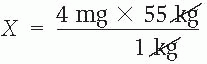Check the zeros and the decimal places

Suppose that you receive an order to administer 0.1 mg of epinephrine subcutaneously, but the only epinephrine on hand is a 1-ml ampule that contains 1 mg of epinephrine. To calculate the volume for injection, use the ratio-andproportion method.

State the problem as a proportion. 1 mg : 1 ml :: 0.1 mg : X Solve for X by applying the principle that the product of the means equals the product of the extremes.

1 ml × 0.1 mg = 1 mg × X

Divide and cancel out the units of measure that appear in both the numerator and the denominator, carefully checking the decimal placement.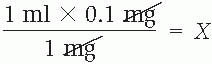Recheck calculations that seem unusual

If, for example, your calculation indicates that you should administer 25 tablets, you’ve probably made an error. Carefully recheck any figures that seem unusual. If you still have doubts, review your calculations with another health care professional.

Determining the number of tablets to administer

Calculating the number of tablets to administer lends itself to the use of ratios and proportions. To perform the calculation, follow this process:

1. Set up the first ratio with the known tablet (tab) strength.

2. Set up the second ratio with the unknown quantity.

3. Use these ratios in a proportion.

4. Solve for X, applying the principle that the product of the means equals the product of the extremes.

For example, suppose that a drug order calls for 100 mg of propranolol P.O. q.i.d., but only 40-mg tablets are available. To determine the number of tablets to administer, follow these steps:

1. Set up the first ratio with the known tablet (tab) strength.

40 mg : 1 tab

2. Set up the second ratio with the desired dose and the unknown number of tablets.

100 mg : X

3. Use these ratios in a proportion.

40 mg : 1 tab :: 100 mg : X

4. Solve for X by applying the principle that the product of the means equals the product of the extremes.

1 tab × 100 mg = 40 mg × X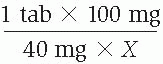Determining the amount of liquid medication to administer

You can also use ratios and proportions to calculate the amount of liquid medication to administer. Simply follow the same four-step process used in determining the number of tablets to administer.

For example, suppose that a patient is to receive 750 mg of amoxicillin oral suspension. The label reads amoxicillin (amoxicillin trihydrate ) 250 mg/5 ml. The bottle contains 100 ml. To determine how many milliliters of amoxicillin solution the patient should receive, follow these steps:

1. Set up the first ratio with the known strength of the liquid medication.

250 mg : 5 ml

2. Set up the second ratio with the desired dose and the unknown quantity.

750 mg : X

3. Use these ratios in a proportion.

250 mg : 5 ml :: 750 mg : X

4. Solve for X by applying the principle that the product of the means equals the product of the extremes.

5 ml × 750 mg = 250 mg × XAdministering drugs available in varied concentrations

Because drugs, such as epinephrine, heparin, and allergy serums, are available in varied concentrations, you must consider the concentration of the drug when calculating a drug dosage. Otherwise, you could make a serious—even lethal—mistake. To avoid a dosage error, make sure that drug concentrations are part of the calculation.

For example, a drug order calls for 0.2 mg of epinephrine subcutaneously stat. The ampule is labeled as 1 ml of 1:1,000 epinephrine. Follow these steps to calculate the correct volume of drug to inject.

1. Determine the strength of the solution based on its unlabeled ratio.

1:1,000 epinephrine = 1 g/1,000 ml

2. Set up a proportion with this information and the desired dose.

1g : 1,000 ml :: 0.2 mg : X

Before you can perform this calculation, however, you must convert grams to milligrams by using the conversion 1g = 1,000 mg.

3. Restate the proportion with the converted units, and solve for X.

1,000 mg : 1,000 ml :: 0.2 mg : X

1,000 ml × 0.2 mg = 1,000 mg × X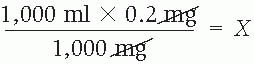0.2 ml = X

Calculating I.V. drip and flow rates

To compute the drip and flow rates, set up a fraction with the volume of solution to be delivered over the prescribed duration. For example, if a patient is to receive 100 ml of solution within 1 hour, the fraction is: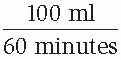Next, multiply the fraction by the drip factor (the number of drops contained in 1 ml) to determine the drip rate (the number of drops per minute to be infused). The drip factor varies among I.V. sets and appears on the package containing the I.V. tubing
administration set. Following the manufacturer’s directions for the drip factor is crucial. Standard sets have drip factors of 10, 15, or 20 gtt/ml. A microdrip (minidrip) set has a drip factor of 60 gtt/ml.

Use the following equation to determine the drip rate:The equation applies to solutions that are infused over many hours as well as to small-volume infusions such as those used for antibiotics, which are given for less than 1 hour.

You can modify the equation by first determining the number of milliliters to be infused over 1 hour (the flow rate). Then, divide the flow rate by 60 minutes. Next, multiply the result by the drip factor to determine the number of drops per minute. You’ll also use the flow rate when working with infusion pumps to set the number of milliliters to be delivered in 1 hour.

Quick calculations of drip rates

In addition to using the equation and its modified version, quicker computation methods are available. To administer solutions ordered at an hourly rate using a microdrip set, adjust the flow rate (ml/hour) to equal the drip rate (gtt/minute). Using the equation, divide the flow rate by 60 minutes and multiply the result by the drip factor, which also equals 60. Because the flow rate and the drip factor are equal, the two arithmetic operations cancel each other out. For example, if the flow rate is 125 ml/hour, the equation would be: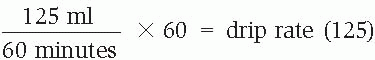Instead of spending the time solving the equation, you can simply use the number assigned to the flow rate as the drip rate. For sets that deliver 15 gtt/ml, the flow rate divided by 4 equals the drip rate. For sets with a drip factor of 10, the flow rate divided by 6 equals the drip rate.

To determine how many micrograms of a drug are in a milliliter of solution, use the following equation:

mcg/ml = mg/ml × 1,000

To express drip rates in micrograms per kilogram per minute (mcg/kg/minute), you must know the concentration of the solution (mcg/ml), the patient’s weight (kg), and the infusion rate (ml/hour):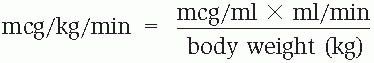To find the milliliters per minute (ml/minute), divide the number of milliliters per hour (ml/hour) by 60.

You can also convert milliliters per hour (ml/hour) from a dosage given in micrograms per kilograms per minute (mcg/kg/min) as follows: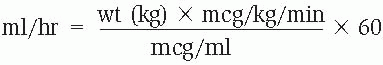Dimensional analysis

Dimensional analysis (also known as factor analysis, or factor labeling) is an alternative method of solving mathematical problems. It eliminates the need to memorize formulas and requires only one equation to determine an answer. To compare the ratio-andproportion method and dimensional analysis at a glance, read the following problem and solutions.

The physician prescribes 0.25 g of streptomycin sulfate I.M. The vial reads “2 ml = 1 g.” How many milliliters should you administer?

Dimensional analysisRatio and proportion

1 g : 2 ml :: 0.25 g : X

2 mg × 0.25 g = 1 g × X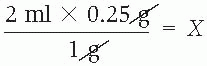Dimensional analysis involves arranging a series of ratios, called factors, into a single fractional equation. Each factor, written as a fraction, consists of two quantities and their units of measurement that are related to each other in a given problem. For instance, if 1,000 ml of a drug should be administered over 8 hours, the relationship between 1,000 ml and 8 hours is expressed by the fraction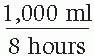When a problem includes a quantity and its unit of measurement that are unrelated to any other factor in the problem, they serve as the numerator of the fraction, and 1 (implied) becomes the denominator.

Some mathematical problems contain all of the information needed to identify the factors, set up the equation, and find the solution. Other problems require the use of a conversion factor. Conversion factors are equivalents (for example, 1 g = 1,000 mg) that can be memorized or obtained from a conversion chart. Because the two quantities and units of measurement are equivalent, they can serve as the numerator or the denominator. Thus, the conversion factor 1 g = 1,000 mg can be written in fraction form as: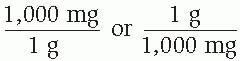The factors given in the problem plus any conversion factors that are necessary to solve the problem are called knowns. The quantity of the answer, of course, is unknown. When setting up an equation in dimensional analysis, work backward, beginning with the unit of measurement of the answer. After plotting all of the knowns, find the solution by following this sequence:

1. Cancel similar quantities and units of measurement.

2. Multiply the numerators.

3. Multiply the denominators.

4. Divide the numerator by the denominator.

Mastering dimensional analysis can take practice, but you may find your efforts well rewarded. To understand more fully how dimensional analysis works, review the following problem and the steps taken to solve it.

The physician prescribes X grains (gr) of a drug. The pharmacy supplies the drug in 300-mg tablets (tab). How many tablets should you administer?

1. Write down the unit of measurement of the answer, followed by an “equal to” symbol (=).

tab =

2. Search the problem for the quantity with the same unit of measurement (if one doesn’t exist, use a conversion factor); place this in the numerator and its related quantity and unit of measurement in the denominator.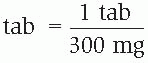Separate the first factor from the next with a multiplication symbol (×).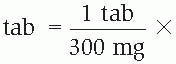Place the unit of measurement of the denominator of the first factor in the numerator of the second factor. Search the problem for the quantity with the same unit of measurement (if there’s no common measurement, as in this example, use a conversion factor). Place this in the numerator and its related quantity and unit of measurement in the denominator; follow with a multiplication symbol. Repeat this step until all known factors are included in the equation.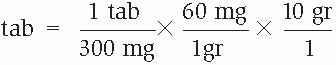Alternatively, you can treat the equation as a large fraction, using these steps.

1. First, cancel similar units of measurement in the numerator and the denominator. What remains should be what you began with—the unit of measurement of the answer; if not, recheck your equation to find and correct the error.

2. Multiply the numerators and then the denominators.

3. Divide the numerator by the denominator.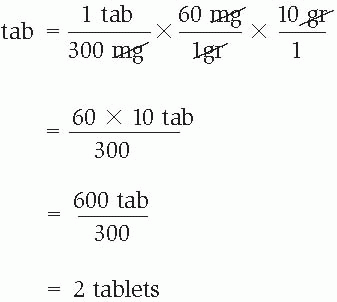= 2 tablets

Indicated for patients with paroxysmal supraventricular tachycardia, including that associated with accessory bypass tracts (Wolff-Parkinson-White syndrome).

Dosage

Initially, give 6 mg I.V. as a rapid bolus over 1 to 3 seconds; if there’s no response in 1 to 2 minutes, give 12 mg I.V. as a rapid bolus (12 mg dose may be given a second time if required).

Nursing considerations

♦ Adverse effects may include flushing or chest pain lasting 1 to 2 minutes.

♦ Flush immediately and rapidly with 20 ml of normal saline solution to ensure drug delivery.

♦ Monitor the cardiac rhythm to detect heart block and transient asystole.

amiodarone

Indicated for recurrent hemodynamically unstable ventricular tachycardia and frequently recurring ventricular fibrillation.

Dosage

Give 150 mg I.V. over the first 10 minutes (15 mg/minute [not to exceed 30 mg/minute]); then 360 mg I.V. over the next 6 hours (1 mg/minute), followed by a maintenance infusion of 540 mg I.V. over the remaining 18 hours (0.5 mg/minute).

Nursing considerations

♦ Administer the drug through a central line whenever possible.

♦ Mix initial 150 mg dose in 100 ml dextrose 5% in water (D5W); the drug is incompatible with normal saline solution. Mix infusions to be administered over 2 hours or longer in glass or polyolefin bottles containing D5W.

♦ Monitor the patient for hypotension, bradycardia, and arrhythmias.

♦ Must be delivered by volumetric infusion pump.

atropine

Indicated for symptomatic sinus bradycardia with hemodynamic compromise, atrioventricular (AV) block, pulseless electrical activity (PEA), and ventricular asystole.

Dosage

For bradycardia or AV block, give 0.5 mg to 1 mg I.V. push, repeated every 3 to 5 minutes to a total dose of 0.04 mg/kg. A total dose of 2.5 mg (0.04 mg/kg) results in full vagal blockade in humans. For asystole or PEA, give 1 mg I.V. push, repeat in 3 to 5 minutes if asystole persists.

Nursing considerations

♦ Monitor the patient for paradoxical initial bradycardia, especially if he’s receiving 0.4 to 0.6 mg.

♦ Monitor the patient’s fluid intake and urine output.

♦ Watch for tachycardia.

dobutamine

Indicated for inotropic support in short-term treatment of adults with cardiac decompensation caused by depressed contractility resulting from organic heart disease or surgery.

Dosage

Give 0.5 to 1 mcg/kg/minute by I.V. infusion, titrating to an optimal dosage of 2 to 20 mcg/kg/minute.

Nursing considerations

♦ Watch for reflex peripheral vasodilation.

♦ Monitor the patient’s heart rate closely; an increase of 10% or more may worsen myocardial ischemia.

♦ Avoid extravasation; give drug through a central venous catheter or a large peripheral vein. Use an infusion pump.

dopamine

Indicated for patients with hypotension or bradycardia, to treat shock, improve perfusion to vital organs, and increase cardiac output.

Dosage

Initially, give 2 to 5 mcg/kg/minute, titrated until the desired response is achieved. Infusion may be increased by 1 to 4 mcg/kg/minute at 10- to 30-minute intervals.

Nursing considerations

♦ Drug effects vary with dosage. At 0.5 to 2 mcg/kg/minute, the drug dilates the renal and mesenteric vessels without increasing heart rate or blood pressure; at 2 to 10 mcg/kg/minute, it increases cardiac output without peripheral vasoconstriction; at more than 10 mcg/kg/minute, it causes peripheral vasoconstriction.

♦ Use a central line or a large vein. Monitor for extravasation; if it occurs, stop the infusion immediately and call the physician.

epinephrine

Indicated for the patient in cardiac arrest.

Dosage

Give 1 mg I.V. or intraosseously repeated every 3 to 5 minutes, if necessary.

Nursing considerations

♦ If administering the drug by the I.V. or intraosseous route isn’t possible, the drug may be given endotracheally at 2 to 2½ times the peripheral I.V. dose.

♦ Intracardiac injection is indicated only during open cardiac massage and if other routes are unavailable.

lidocaine

Indicated for patients with ventricular tachycardia (VT), ventricular ectopy, and ventricular fibrillation (VF); prophylactic administration in patients with uncomplicated myocardial infarction isn’t recommended.

Dosage

Give 1 to 1.5 mg/kg I.V. bolus as a loading dose at 25 to 50 mg/minute; repeat the bolus dose every 3 to 5 minutes until arrhythmias subside or adverse reactions develop, to a maximum of 3 mg/kg (300 mg over 1 hour); simultaneously, set up a continuous I.V. infusion at 1 to 4 mg/minute. (Dilute 1 g of lidocaine in 250 ml of dextrose 5% in water for a 0.4% solution, or 4 mg/ml.)

Nursing considerations

♦ For an elderly patient, a patient weighing less than 110 lb (50 kg), or a patient with heart failure or hepatic disease, administer half of the bolus dose.

♦ Lidocaine improves the response to defibrillation when the patient is in VF.

magnesium sulfate

Indicated for the treatment of ventricular fibrillation, ventricular tachycardia, cardiac arrest associated with torsades de pointes, and torsades de points with pulses.

Dosage

Give 1 to 2 g in 10 ml of dextrose 5% in water (D5W) administered I.V. or intraosseously over 5 to 20 minutes for cardiac arrest associated with torsades de pointes. Give 1 to 2 g in 50 to 100 ml
of D5W I.V. over 5 to 60 minutes and follow this with 0.5 to 1 g/hour I.V. for torsades de pointes with pulses.

Nursing considerations

♦ Monitor serum magnesium levels.

♦ Watch for respiratory depression and signs and symptoms of heart block.

♦ If hypotension develops, slow or stop the infusion.

nitroglycerin

Indicated for patients with heart failure associated with myocardial infarction and for patients with unstable angina.

Dosage

Give a 5 mcg/minute I.V. infusion initially, increasing by 5 mcg/minute every 3 to 5 minutes until a response occurs. If a 20 mcg/minute rate doesn’t produce a response, increase the dosage by as much as 20 mcg/minute every 3 to 5 minutes. Up to 100 mcg/minute may be needed.

Nursing considerations

♦ Monitor the patient for hypotension, which could worsen myocardial ischemia.

♦ Because up to 80% of the drug binds to the plastic in normal I.V. administration sets, use the special I.V. tubing supplied by the manufacturer.

♦ Mix in glass bottles and avoid using I.V. filters. Use an infusion pump.

nitroprusside

Indicated for the patient with heart failure or in hypertensive crisis.

Dosage

Dissolve 50 mg in 2 to 3 ml of dextrose 5% in water (D5W); then mix with 250 to 1,000 ml of D5W, depending on the desired concentration. Begin the infusion at 0.3 mcg/kg/minute and titrate every few minutes to a maximum of 10 mcg/kg/minute.

Nursing considerations

♦ Wrap the container in opaque material to prevent deterioration of the drug.

♦ Monitor the patient’s blood pressure every 5 minutes for 1 hour and every 15 minutes thereafter.

♦ Large doses given at fast infusion rates increase the risk of cyanide toxicity. Measure cyanide levels and monitor for acidosis. If a toxic reaction is suspected, start treatment without the test results.

norepinephrine bitartrate

Indicated for the patient with severe hypotension or low total peripheral resistance.

Dosage

Initially, give an 8 to 12 mcg/minute I.V infusion. Titrate to maintain systolic blood pressure at 80 to 100 mm Hg in a previously normotensive patient. The average rate of maintenance infusion is 2 to 4 mcg/minute.

Nursing considerations

♦ Norepinephrine is contraindicated in the patient with hypovolemia.

♦ Monitor the patient’s blood pressure with an intra-arterial line because measurements obtained with a standard cuff may be falsely low.

♦ Cardiac output may increase or decrease, depending on vascular resistance, left ventricular function, and reflux response.

♦ Avoid prolonged use; the drug may cause ischemia of vital organs.

♦ Use a central venous catheter or a large vein to minimize the risk of extravasation. If extravasation occurs, stop the infusion and call the physician.

procainamide

Indicated for the patient with ventricular arrhythmias, such as premature ventricular contractions or tachycardia, when lidocaine is contraindicated or ineffective.

Dosage

Give a loading dose infusion of 20 mg/minute, not to exceed a total dose of 500 mg, followed by a continuous infusion of 2 to 6 mg/minute.

Nursing considerations

♦ Lower the dosage as ordered, for the patient with renal failure.

♦ Rapid infusion will cause acute hypotension. An infusion pump is required.

♦ Monitor electrocardiogram results carefully; if the QRS complex widens more than 50% or if the QT interval is prolonged, notify the physician and discontinue the infusion, as ordered.

vasopressin

Indicated for the treatment of adult shock-refractory ventricular fibrillation, asystole, or pulseless electrical activity.

Dosage

Give 40 units I.V., one time only.

Nursing considerations

♦ Use as an alternative to epinephrine.

Aug 18, 2016 | Posted by in NURSING | Comments Off on Dosage calculationEnsuring effective therapy## Full access? Get Clinical TreeGet Clinical Tree app for offline access